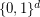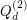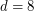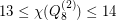# Coloring squares of hypercubes (Solved)

 Importance: Medium ✭✭
 Author(s): Wan, Peng-Jun
 Subject: Graph Theory » Coloring » » Vertex coloring
 Keywords: coloring hypercube
 Recomm. for undergrads: yes
 Posted by: mdevos on: November 21st, 2007
 Solved by: See pgregor's reference

Ifis a simple graph, we letdenote the simple graph with vertex setand two vertices adjacent if they are distancein.

Conjecture.

Here, denotes the-dimensional hypercube, i.e. the graph with vertex setand two vertices adjacent if they differ in exactly one coordinate. So, the vertices with at most one coordinate equal toform a clique of sizein, giving us the easy lower bound. An independent set inis precisely an error correcting code, so a proper coloring of this graph may be viewed as a covering of the hypercube with codes. Wan [W] constructed a coloring ofusingmany colors, and he conjectures that this is optimal.

Note thatcontains a subgraph isomorphic to, so it suffices to prove the conjecture whenis a power of 2. The casesare rather easy to verify, butappears to be open.

In his Ph.D. thesis, Ghebleh suggests the stronger conjecture thathas circular chromatic number equal to.

## Bibliography

[G] M. Ghebleh, Theorems and Computations in Circular Colourings of Graphs, Ph.D. thesis, Simon Fraser University, 2007.

*[W] P. J. Wan, Near-optimal conflict-free channel set assignments for an optical cluster-based hypercube network. J. Comb. Optim. 1 (1997), no. 2, 179--186. MathSciNet.

* indicates original appearance(s) of problem.

### disproved

Actually, the conjecture is disproved by, obtained independently by Hougardy in 1991  and Royle in 1993 [2, Section 9.7]. Moreover, although not fully determined, it is known thatasymptotically attains the lower bound, as, proven by Ostergard in 2004 .

There are also several results onfor general, and in particular on[3,4].

 G.M. Ziegler, Coloring Hamming graphs, optimal binary codes, and the 0/1 Borsuk problem in low dimensions, in: H. Alt (Ed.), Computational Discrete Mathematics, Springer, Berlin, 2001, 159-171.

 T.R. Jensen, B. Toft, Graph Coloring Problems, Wiley, New York, 1995.

 P.R.J. Ostergard, On a Hypercube coloring problem, J. Combin. Theory A 108 (2004), 199-204.

 H.Q. Ngo, D.-Z. Du, R.L. Graham, New bounds on a hypercube coloring problem, Inform. Process. Lett. 84 (2002), 265-269.

## Comment viewing options

Select your preferred way to display the comments and click "Save settings" to activate your changes.